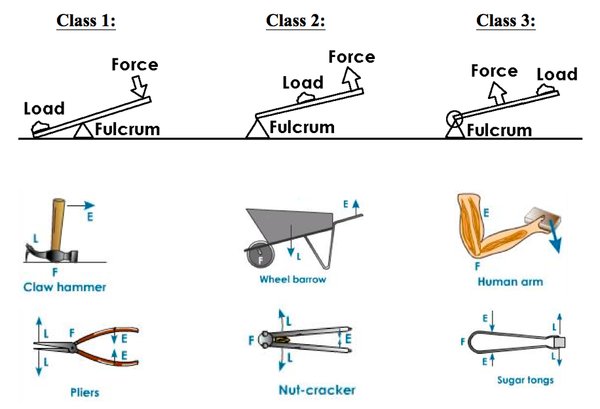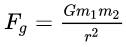# Top 15 Examination Question And Answer On Physics For Secondary School Students

Physics (from Ancient Greek: φυσική (ἐπιστήμη)translit. physikḗ (epistḗmē)lit. ‘knowledge of nature’, from φύσις phýsis” nature” is the natural science that studies matter and its motion and behavior through space and time and that studies the related entities of energy and force. Physics is one of the most fundamental scientific disciplines, and its main goal is to understand how the universe behaves. In this article, we are considering the top 15 examination question and answer on physics for secondary school students.

## 1.Question:

Who invented physics?

No one knows who the first physicist was, but the Sumerians and Ancient Egyptians both developed a basic understanding of some rudimentary concepts in physics nearly 5000 years ago. The ancient Greeks also made some major discoveries in the field of physics, and, in fact, the word physics derived from Ancient Greek. For instance, Democritus (c. 460 – 370 BCE) was the first to theorize that matter was composed of atoms.

## 2.Question:

What is quantum physics?

Quantum physics is more commonly referred to as quantum mechanics. It is an attempt by scientists to understand the universe but on a very, very small scale. The idea is that if we can understand the smallest particles we can find, we might be able to gather a larger understanding of the universe as a whole.

## 3.Question:

What are three ways an object can accelerate?

There are three ways an object can accelerate: a change in velocity, a change in direction, or a change in both velocity and direction.

Imagine a racecar that’s traveling in a straight line. If it changes velocity (speeds up or slows down), then it’s accelerating. This type of acceleration, with only a change in velocity but no change in direction, is called linear acceleration. If the racecar is instead driving around a circular track at a constant speed, only its direction is changing. This kind of acceleration is called centripetal or radial acceleration. If the car changed both its velocity and its direction, then we would have angular acceleration.

## 4.Question:

Is there any proof to the 2nd law of thermodynamics?

The second law of thermodynamics usually happens and can be observed in real life. Since that is the case, there are a lot of proofs, which you can consider, for it. Some of those are: When you make a cup of coffee. You will notice how it will get colder until it reaches room temperature. You see, water or your coffee in this sense cannot spontaneously heat up. When you fart, it doesn’t just stay in one place. It continuously spreads into the whole room. The reason behind it is how heat, gas particles and energy moves from high concentration areas to areas that have a low concentration. And that is what the second law of thermodynamics is all about.

## 5.Question:

What do all waves have in common?

When looking at sound, light, and even water waves, all waves contain energy. This energy is in the form of repetitive vibration, such as an up and down movement or a side to side movement. In any case, this energy can move from one spot to another, either through a medium like water or through a vacuum like space.

Energy is measured by taking Planck’s constant, which is 6.63 x 10 ^-34 J per second, and multiplying it by the frequency of the wave. Usually, the higher the frequency, the more energy the wave has. If the wave continues through a medium and the frequency becomes less and less, the energy becomes less and less.

## 6.Question:

How much does a marble weigh?

A marble weighs nearly 4-5 gm.

Marbles are often made with glass or plastic, and are usually lightweight and used as toys or for decoration. Moreover, 1 gm is equal to 0.035 ounces. Therefore, a 4 or 5 gm marble is 0.141 or 0.176 ounces.

## 7.Question:

A 60 kg student is standing atop a spring in an elevator that is accelerating upward at 3.0 m/s2. The spring constant is 2.5 x 103 N/m. By how much is the spring compressed?

Given the mass of the student, m = 60 kg, and the acceleration of the elevator, a = 3.0 m/s2, let the spring be compressed by a length x meter. Then there are two forces acting on the student. One is his own weight W acting downward and the other is the spring force S by the compression of the spring acting upward. Note that a spring with spring constant k and compression x exerts a force of S = k ∗ x, in a direction opposite to the compression.

## 8.Question:

A hot air balloon begins its descent at a rate of 22 1/2 feet per minute. how long will it take for balloons elevation to change by -315 feet? During another part of its flight, the balloon in above had a change in elevation of -901 ft. What was its rate of descent?

For the first problem, the change in elevation = -315 ft. The rate of descent = -22(1/2) ft/min (Please note that the balloon is descending and thus we have used a negative sign for the rate of change where a positive sign will imply an ascent) =-45/2 ft/min. As you may observe that rate of descent is nothing but the velocity of the balloon and thus the time taken may be evaluated by using, time=distance/speed =(-315)/(-45/2) min =(-315*2)/(-45) min =7*2 min =14 min. Thus time taken for balloon to descend is 14 min. In the next problem, you require the rate of descent which may be found by using, speed=distance/time. Change in elevation of balloon= -901 ft. Rate of descent= (-901)/time. Here we do not need the time taken by balloon and thus we’re unable to calculate the rate of descent. Once we have the time for flight, we’ll plug it in the equation above to get the rate of descent.

## 9.Question:

How is work, force, and distance related?

Work is a measure of energy transfer when force is applied in a certain direction. It is measured in joules. Work is related to force and distance by the mathematical equation: work = force x distance.

One could understand this equation as 1 joule of work has occurred when 1 newton of force created a displacement of 1 meter. Force, in this equation, usually refers to the weight of the object given by mass x gravity. The equation for work also implies that a small displacement of a very heavy object would be the same work as a small, lighter object moved a long distance.

## 10.Question:

A wheelbarrow is an example of what type of lever?

Wheelbarrows are a type of lever. There are three main types of levers and a wheelbarrow in the second class. Let’s take a look at the three classes of levers.

#### First Class Levers

The position of the fulcrum in this class of levers lies between the effort and the resistance. The effort is applied at one end of the lever in order to move the weight on an opposite side. Common first-class levers are see-saws and pliers.

#### Second Class Levers

The position of the fulcrum in this class of levers lies at one end of the lever while the effort is on the opposite end. In this type of lever, the weight is carried in the middle. Some common second class levers are wheelbarrows and doors.

#### Third Class Levers

The position of the fulcrum in this class of levers lies at one end of the lever while the effort lies in the middle and the weight is on the opposite end. More energy is needed in this class of lever, but a greater distance of movement is seen. Some common third class levers are a baseball bat and our human arms.## 11.Question:

How are evaporation and sublimation similar?

Evaporation and sublimation are similar because they both are phase changes where the substance turns into a gas. Although they both end in the gas stage, they don’t begin with the same substance. Evaporation is when a liquid turns into a gas, while sublimation is when a solid turns into a gas. Both involve absorbing energy to break apart the bonds of the substance.

An example of evaporation is steam coming from a boiling pot. The liquid water is being heated enough that the bonds between the water molecules break, forming gas molecules. On the other hand, an example of sublimation is dry ice. If left out, dry ice will turn from a solid to a gas, without hitting the liquid stage. This may seem quite strange to the viewer, but the dry ice goes straight to a gas because carbon dioxide at room temperature is a gas.

## 12.Question:

The gravitational force between two objects increases as mass?

The gravitational force between two objects increases as mass increases.

The universal law of gravitation is an equation that quantifies the force that exists between any two masses. This equation is:• Fg is the force of gravity between the masses. Force is measured in newtons (N).
• G is the universal gravitational constant (6.67 x 10-11 Nm2/kg2).
• m1 and m2 are the masses between which the force is being calculated. The unit for mass in kilograms (kg).
• r is the distance between the centers of each mass in meters (m).

We can see from this equation that the masses are multiplied and are directly proportional to the gravitational force. This means if the mass of either or both masses increases, the force increases. If the distance between the masses increases the force between them decreases by 1/r2.

## 13.Question:

Why is breaking glass a physical change?

Breaking glass is an example of a physical change because if you break it, it is still glass. It didn’t change to another substance. It still has the same atoms and molecules, but its shape has changed.

Another example of this is tearing paper into pieces. It is a physical change because it is still paper.

## 14.Question:

The answer to: What is the buildup of electric charges on an object?